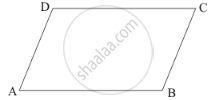Advertisement Remove all ads

# Complete the Following Statement by Means of One of Those Given in Brackets Against Each: If Opposite Angles of a Quadrilateral Are Equal, Then It is Necessarily a - Mathematics

MCQ
Fill in the Blanks

Complete the following statement by means of one of those given in brackets against each:

If opposite angles of a quadrilateral are equal, then it is necessarily a ....................

#### Options

• parallelogram

•  rhombus

•  rectangle

Advertisement Remove all ads

#### Solution

If opposite angles of a quadrilateral are equal, then it is necessarily a parallelogram.

Reason:ABCD is a quadrilateral in which  ∠A =∠C and ∠B = ∠D.

We need to show that ABCD is a parallelogram.

In quadrilateral ABCD, we have

∠A = ∠C

∠B = ∠D

Therefore,

∠A + ∠B = ∠C + ∠D …… (i)

Since sum of angles of a quadrilateral is  360°

∠A + ∠B = ∠C + ∠D = 360°

From equation (i), we get:

(∠A + ∠B) + ( ∠A + ∠B) = 360°

2(∠A +∠B ) = 360°

∠A + ∠B =  180°

Similarly,  ∠C + ∠D = 180°

Now, line AB intersects AD and BC at A and B respectively

Such that  ∠A +∠B = 180°

That is, sum of consecutive interior angles is supplementary.

Therefore, .AD || BC

Similarly, we get AB || DC.

Therefore, ABCD is a parallelogram.

Is there an error in this question or solution?
Advertisement Remove all ads

#### APPEARS IN

RD Sharma Mathematics for Class 9
Chapter 13 Quadrilaterals
Q 14.7 | Page 69
Advertisement Remove all ads
Advertisement Remove all ads
Share
Notifications

View all notifications

Forgot password?
Course